Showing posts with label L5. Show all posts
Showing posts with label L5. Show all posts

## Saturday, November 10, 2012

### ArtemisIllustration of Artemis-P1 liberations orbits. Credit: NASA/Goddard

ARTEMIS-P1 is the first spacecraft to navigate to and perform stationkeeping operations around the Earth-Moon L1 and L2 Lagrangian points. There are five Lagrangian points associated with the Earth-Moon system. The two points nearest the moon are of great interest for lunar exploration. These points are called L1 (located between the Earth and Moon) and L2 (located on the far side of the Moon from Earth), each about 61,300 km (38,100 miles) above the lunar surface. It takes about 14 to 15 days to complete one revolution about either the L1 or L2 point. These distinctive kidney-shaped orbits are dynamically unstable and require weekly monitoring from ground personnel. Orbit corrections to maintain stability are regularly performed using onboard thrusters.

## Sunday, March 01, 2009

### Gravity Wave Spectrum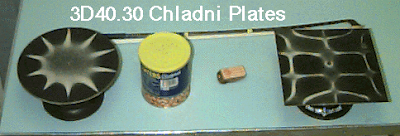PURPOSE: To show the two-dimensional standing waves on the surface of a square or circular plate.

Early perception of sound as analogy to the ideas of the WMAP background were forming in my mind when Wayne HU was demonstrating the image of polarizations in B mode. To me its as if one puts on a pair of glasses and based on an assumption of the gravitational waves, then one tends to see "all of it" in this Lagrangian way.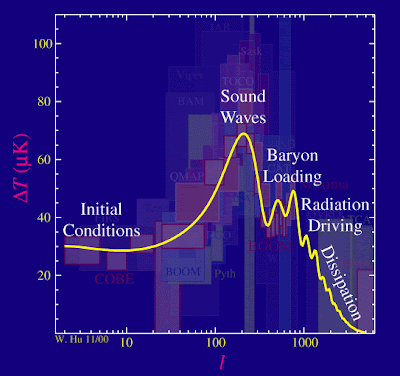With the discovery of sound waves in the CMB, we have entered a new era of precision cosmology in which we can begin to talk with certainty about the origin of structure and the content of matter and energy in the universe.Polarization

This was the basis of how I was seeing the progression of Webber's experiments in using the aluminum bars in gravitational wave detection. It was also more then this that I came to the conclusion I did.

***

Sounding out the Big BangJun 1, 2007 by Craig J Hogan is in the departments of physics and astronomy at the University of Washington, Seattle, US.

Our view of the universe is about to change forever. Since science began, all our knowledge of what lies above, below and around us has come from long-familiar forms of energy: light, produced by distant astrophysical objects; and matter, in the form of particles such as cosmic rays. But we are now in a position to study the universe using an entirely different form of energy that until now has never been directly detected – gravitational waves.Gravitational waves open up a new window on the universe that will allow us to probe events for which no electromagnetic signature exists. In the next few years, the ground-based interferometers GEO-600, LIGO, VIRGO and TAMA should be able to detect the high-frequency gravitational waves produced by extreme astrophysical objects, providing the first direct detection of these disturbances in space–time. With its much longer arm lengths, the space-based interferometer LISA will, if launched, be able to detect lower-frequency gravitational waves, possibly those generated by phase transitions in the early universe. At even lower frequencies, other experiments will look for tiny signatures of gravitational waves in the cosmic microwave background. Source: NASA.The flow of energy in a cosmic phase transition is similar to that in a waterfall, with turbulence in the cosmic fluid generating a gravitational-wave background today.

***

Sound and fluidized interpretation seemed very close to me of the way in which such analogy would help us to look at the universe and the spaces in between cosmological locations, as if, in a three body problem relation.

The Origin of the Universe as Revealed Through the Polarization of the Cosmic Microwave Background submitted by Scott Dodelson Sun, 22 Feb 2009 14:27:37 GMT

Modern cosmology has sharpened questions posed for millennia about the origin of our cosmic habitat. The age-old questions have been transformed into two pressing issues primed for attack in the coming decade: How did the Universe begin? and What physical laws govern the Universe at the highest energies? The clearest window onto these questions is the pattern of polarization in the Cosmic Microwave Background (CMB), which is uniquely sensitive to primordial gravity waves. A detection of the special pattern produced by gravity waves would be not only an unprecedented discovery, but also a direct probe of physics at the earliest observable instants of our Universe. Experiments which map CMB polarization over the coming decade will lead us on our first steps towards answering these age-old questions.

***

See:
• Sound Waves in the CMB
• The Sound of the Landscape
• Distinctions of Holographical Sound
• B Field Manifestations
• ## Friday, October 03, 2008

### Centroids

Conclusion:The state of mind of the observer plays a crucial role in the perception of time.Einstein

It is often I consider the Center of Gravity(Mass) as an illustration of the perspective that exists "around and within us" as a product of the natural world. We are limited by our own knowledge, yet when given, and lead to observation of what is natural in our world, we see where we had not seen before.

\bar z = \frac{\int z dm}{m}The center of gravity (or mass), abbreviated as COM, of any object is that point within the object upon which gravity (or any body force) acts, regardless of the orientation of the object. The COM of an object may be calculated by using the principle of equilibrium.

First off you must know that I work according to a set of principles that were discovered after a time through "subjective reflection" on the nature of mind and how it works. Of course I am no expert here, but the principles themself are realizable as an active function in the recognition of how one might interpret the world.

\bar y = \frac{\int y \rho dV}{\int \rho dV}In the event that the density ρ of an object is not uniform throughout, the calculation of COM may be done by a similar set of equations involving the addition of density to the analysis.

It is by taking one to recognisance of the title of this blog entry that I wanted to discuss the "subjective part" and the understanding of the "mathematical construct" that one, in my opinion exists. Just as we progress to understand the natural world around us, I believe these are synonymous with each other.

\bar Y = \frac{\sum m \bar y}{\sum m}If a body is made up of multiple sections, each of which has a unique mass, the method for evaluating the centroid of that body is to evaluate the composite body by finite element analysis of each of the sections through the use of moment balancing, as above.

Liminocentric structuresYou can find much on this site in regards to this issue, and I now relate centroid for consideration in the case of this topic. I presented it at the Backreaction site today to show an advancement in the thinking as we move to incorporate the knowledge. As we come to understand the natural world around us.

Giving earth a new understanding in terms of its densities and it's relation to the gravitational contribution, it has in our new views of the globe, helped me learn and understand the feature of gravity as it extends to the cosmos.

I have taken it a step further as many of you know to include the emotive states as valued physiological relations to what manifests in the immediate environs of the body home. While this is a far cry from the understanding of gravitational research it was inevitable for me to see an extension of how one can topological relate to the world, in this inductive/deductive mode to reason.

While living on this earth, we always come home for reflection, and if any have not taken stock of what has accumulated during the day, then it is inevitable that is will come to reside very close to home as one sleeps through the nocturnality night. This research of subjectivity and mind had to entail "all of our activities." It had to include an understanding of the "mathematical constructs" that we observe, in order to relate to nature.

List of Centroids

ShapeFigure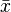$\bar x$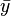$\bar y$Area
Triangular area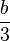$\frac{b}{3}$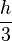$\frac{h}{3}$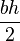$\frac{bh}{2}$
Quarter-circular area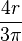$\frac{4r}{3\pi}$$\frac{4r}{3\pi}$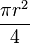$\frac{\pi r^2}{4}$
Semicircular area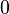$\,\!0$$\frac{4r}{3\pi}$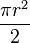$\frac{\pi r^2}{2}$
Quarter-elliptical area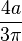$\frac{4a}{3\pi}$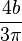$\frac{4b}{3\pi}$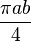$\frac{\pi a b}{4}$
Semielliptical areaThe area inside the ellipse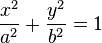$\frac{x^2}{a^2} + \frac{y^2}{b^2} = 1$ and above the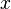$\,\!x$ axis$\,\!0$$\frac{4b}{3\pi}$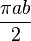$\frac{\pi a b}{2}$
Semiparabolic areaThe area between the curve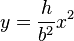$y = \frac{h}{b^2} x^2$ and the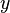$\,\!y$ axis, from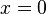$\,\!x = 0$ to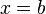$\,\!x = b$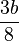$\frac{3b}{8}$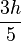$\frac{3h}{5}$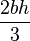$\frac{2bh}{3}$
Parabolic areaThe area between the curve$\,\!y = \frac{h}{b^2} x^2$ and the line$\,\!y = h$$\,\!0$$\frac{3h}{5}$$\frac{4bh}{3}$
Parabolic spandrelThe area between the curve$\,\!y = \frac{h}{b^2} x^2$ and the$\,\!x$ axis, from$\,\!x = 0$ to$\,\!x = b$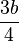$\frac{3b}{4}$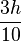$\frac{3h}{10}$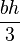$\frac{bh}{3}$
General spandrelThe area between the curve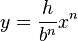$y = \frac{h}{b^n} x^n$ and the$\,\!x$ axis, from$\,\!x = 0$ to$\,\!x = b$$\frac{n + 1}{n + 2} b$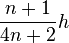$\frac{n + 1}{4n + 2} h$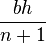$\frac{bh}{n + 1}$
Circular sectorThe area between the curve (in polar coordinates)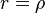$\,\!r = \rho$ and the pole, from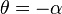$\,\!\theta = -\alpha$ to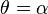$\,\!\theta = \alpha$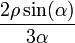$\frac{2\rho\sin(\alpha)}{3\alpha}$$\,\!0$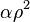$\,\!\alpha \rho^2$
Quarter-circular arcThe points on the circle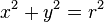$\,\!x^2 + y^2 = r^2$ and in the first quadrant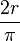$\frac{2r}{\pi}$$\frac{2r}{\pi}$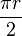$\frac{\pi r}{2}$
Semicircular arcThe points on the circle$\,\!x^2 + y^2 = r^2$ and above the$\,\!x$ axis$\,\!0$$\frac{2r}{\pi}$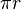$\,\!\pi r$
Arc of circleThe points on the curve (in polar coordinates)$\,\!r = \rho$, from$\,\!\theta = -\alpha$ to$\,\!\theta = \alpha$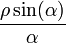$\frac{\rho\sin(\alpha)}{\alpha}$$\,\!0$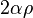$\,\!2\alpha \rho$

Why are Planets Round?

It is always interesting to see water in space.Image: NASA/JPL-
Planets are round because their gravitational field acts as though it originates from the center of the body and pulls everything toward it. With its large body and internal heating from radioactive elements, a planet behaves like a fluid, and over long periods of time succumbs to the gravitational pull from its center of gravity. The only way to get all the mass as close to planet's center of gravity as possible is to form a sphere. The technical name for this process is "isostatic adjustment."

With much smaller bodies, such as the 20-kilometer asteroids we have seen in recent spacecraft images, the gravitational pull is too weak to overcome the asteroid's mechanical strength. As a result, these bodies do not form spheres. Rather they maintain irregular, fragmentary shapes.

See:Isostatic Adjustment is Why Planets are Round?